Next: Regularity of parametric matrices Up: Linear algebra Previous: Linear algebra   Contents

### Enclosure of an interval linear system

Let consider the family of linear systems defined by the matrix equality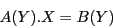where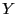is a set of unknowns,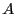a square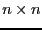matrix whose elements are functions ofand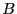a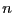dimensional vector whose elements are also functions of. The enclosure of the set of solutions of this family of linear systems is a box that includes the solution of all linear systems in the family.

The enclosure can be computed using the LinearBound procedure whose syntax is


LinearBound(A,B,Derivative,Vars,Init)

where:
• A is a square array whose elements are function of the unknowns in Vars
• B is an array whose elements are function of the unknowns in Vars
• Derivative is an integer. If set to 0 the procedure uses the classical interval Gaussian elimination scheme to calculate the enclosure. If set to 1 it will use an ALIAS specific version of the Gaussian elimination scheme that uses the derivatives of the elements of A, B to improve the enclosure calculation
• Vars: a list of unknowns names
• Init: a list of ranges for the unknowns
A typical example is:

with(ALIAS):
with(linalg):

A:=array([[x,y],[x,x]]):
B:=array([x,y]):
VAR:=[x,y]:
LinearBound(A,B,0,[x,y],[[3,4],[1,2]]);
LinearBound(A,B,1,[x,y],[[3,4],[1,2]]);

which returns the enclosure [[.76923076923077, 10], [-13, -.076923076923077]] if Derivative is set to 0 and [[.91666666666667, 2.6666666666667], [-2, -.66666666666667]] if Derivative is set to 1 (note that these values are computer dependent), the exact answer being [[1.25,1.66666],[-1]].Next: Regularity of parametric matrices Up: Linear algebra Previous: Linear algebra   Contents
Jean-Pierre Merlet 2012-12-20